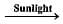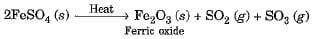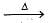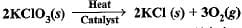NTSE Level Test: Chemical Reactions & Equations

# NTSE Level Test: Chemical Reactions & Equations - Class 10

Test Description

## 20 Questions MCQ Test Online MCQ Tests for Class 10 - NTSE Level Test: Chemical Reactions & Equations

NTSE Level Test: Chemical Reactions & Equations for Class 10 2023 is part of Online MCQ Tests for Class 10 preparation. The NTSE Level Test: Chemical Reactions & Equations questions and answers have been prepared according to the Class 10 exam syllabus.The NTSE Level Test: Chemical Reactions & Equations MCQs are made for Class 10 2023 Exam. Find important definitions, questions, notes, meanings, examples, exercises, MCQs and online tests for NTSE Level Test: Chemical Reactions & Equations below.
Solutions of NTSE Level Test: Chemical Reactions & Equations questions in English are available as part of our Online MCQ Tests for Class 10 for Class 10 & NTSE Level Test: Chemical Reactions & Equations solutions in Hindi for Online MCQ Tests for Class 10 course. Download more important topics, notes, lectures and mock test series for Class 10 Exam by signing up for free. Attempt NTSE Level Test: Chemical Reactions & Equations | 20 questions in 20 minutes | Mock test for Class 10 preparation | Free important questions MCQ to study Online MCQ Tests for Class 10 for Class 10 Exam | Download free PDF with solutions
 1 Crore+ students have signed up on EduRev. Have you?
NTSE Level Test: Chemical Reactions & Equations - Question 1

### Mark the incorrect statement :

Detailed Solution for NTSE Level Test: Chemical Reactions & Equations - Question 1

“The statement that substances appearing on the left hand side of the equation are called products” is incorrect.

NTSE Level Test: Chemical Reactions & Equations - Question 2

### 2AgI(s)2Ag(s) + I2(g) The colour of iodine is

Detailed Solution for NTSE Level Test: Chemical Reactions & Equations - Question 2

Iodine is purple in colour.

NTSE Level Test: Chemical Reactions & Equations - Question 3

### What is the number of iron atoms and hydrogen atoms in the balanced equation for the reaction of steam on iron ?

Detailed Solution for NTSE Level Test: Chemical Reactions & Equations - Question 3

The balanced equation for the reaction is
3Fe + 4H2O → Fe3O4 + 4H2

NTSE Level Test: Chemical Reactions & Equations - Question 4

MnO2 + 4HCI→ MnCl2 + H2O + Cl2 The oxidising agent is

Detailed Solution for NTSE Level Test: Chemical Reactions & Equations - Question 4

In the reaction MnO2 + 4HCl -> MnCl2 + Cl2 + 2H2O , Cl (chlorine) is the substance which is oxidized from the HCl, and it is the reducing agent for the MnO2 as well. As Cl is the oxidized substance in HCl, thus it is the oxidizing agent in the reaction.

NTSE Level Test: Chemical Reactions & Equations - Question 5

The shiny finish to the walls is because of

Detailed Solution for NTSE Level Test: Chemical Reactions & Equations - Question 5

The calcium hydroxide used for white washing the walls reacts with the carbon dioxide (CO2) present in the air to form Calcium carbonate (CaCO3) which gives shiny appearance to the walls.
The chemical reaction occurring during the conversion of slaked lime (calcium hydroxide) to calcium carbonate which gives the shiny appearance to the walls is as follows:
Ca(OH)2 + CO2 → CaCO3 + H2O

NTSE Level Test: Chemical Reactions & Equations - Question 6

Zinc reacts with silver nitrate to form which compounds?

Detailed Solution for NTSE Level Test: Chemical Reactions & Equations - Question 6

Zn + 2AgNO3→ Zn(NO3)2 + 2Ag

NTSE Level Test: Chemical Reactions & Equations - Question 7

On heating ferrous sulphate crystals, we obtain

Detailed Solution for NTSE Level Test: Chemical Reactions & Equations - Question 7

The reaction takes place as underNTSE Level Test: Chemical Reactions & Equations - Question 8

The brown gas evolved on heating of copper nitrate is

Detailed Solution for NTSE Level Test: Chemical Reactions & Equations - Question 8

2Cu(NO3)22CuO + 4NO2 + O2

NTSE Level Test: Chemical Reactions & Equations - Question 9

Which of the following are exothermic processes ?
(i) Reaction of water with quick lime
(ii) Dilution of an acid
(iii) Evaporation of water
(iv) Sublimation of camphor (crystals)

Detailed Solution for NTSE Level Test: Chemical Reactions & Equations - Question 9

Heat is evolved only when water reacts with quick lime and when the acid is diluted with water.

NTSE Level Test: Chemical Reactions & Equations - Question 10

In which of the following chemical equations, the abbreviations represent the correct states of the reactants and products involved at reaction temperature?

Detailed Solution for NTSE Level Test: Chemical Reactions & Equations - Question 10

As we know, H2 and O2 are present in the form of gas in atmosphere and H2O as liquid.

NTSE Level Test: Chemical Reactions & Equations - Question 11

The reaction 4NH3 (g) + 5O2(g) → 4NO(g) + 6H2O(g) is an example of a
(i) Displacement reaction.
(ii) Combination reaction.
(iii) Redox reaction.
(iv) Neutralisation reaction.

Detailed Solution for NTSE Level Test: Chemical Reactions & Equations - Question 11

4NH3 (g) + 5O2(g) → 4NO(g) + 6H2O(g) is an example of displacement as well as redox reaction. Oxygen displaces hydrogen of ammonia. Hence, this is a displacement reaction. Nitrogen of ammonia is getting oxidized with the addition of oxygen. This is also an example of redox reaction.

NTSE Level Test: Chemical Reactions & Equations - Question 12

Barium chloride on reacting with ammonium sulphate forms barium sulphate and ammonium chloride. Which of the following correctly represents the type of the reaction involved
(i) Displacement reaction
(ii) Precipitation reaction
(iii) Combination reaction
(iv) Double displacement reaction

Detailed Solution for NTSE Level Test: Chemical Reactions & Equations - Question 12

BaCl2​ + (NH4​)2​SO4​ →  BaSO4​ + 2NH4​Cl
Above reaction is a double displacement reaction as ions (both cations and anions) of the reacting molecules are exchanged. Barium ion (Ba2+) combines with sulphate ion (SO42−​) to form Barium sulphate (BaSO4​) and ammonium ion (NH4+​) combines with chloride ion(Cl) ion to form ammonium chloride(NH4​Cl).
The above reaction is also classified as a precipitation reaction because barium and sulphate ions combine to form precipitates of barium sulphate.
Ba2+ + SO42−​→ BaSO4​(s)

NTSE Level Test: Chemical Reactions & Equations - Question 13

Which of the following is (are) an endothermic process(es) ?
(i) Dilution of sulphuric acid
(ii) Sublimation of dry ice
(iii) Condensation of water vapours
(iv) Evaporation of water

Detailed Solution for NTSE Level Test: Chemical Reactions & Equations - Question 13

Heat is absorbed when dry ice sublimes and water evaporates. Therefore, cooling is produced.

NTSE Level Test: Chemical Reactions & Equations - Question 14

The following reaction is used for the preparation of oxygen gas in the laboratoryWhich of the following statement (s) is (are) correct about the reaction ?

Detailed Solution for NTSE Level Test: Chemical Reactions & Equations - Question 14

Clearly, It is a decomposition reaction and it requires heat to get decomposed into KCl and O2.

NTSE Level Test: Chemical Reactions & Equations - Question 15

Solid calcium oxide reacts vigorously with water to form calcium hydroxide accompanied by liberation of heat. This process is called slaking of lime. Calcium hydroxide dissolves in water to form its solution called lime water. Which among the following is (are) true about slaking of lime and the solution formed ?
(i) It is an endothermic reaction.
(ii) It is an exothermic reaction.
(iii) The pH of the resulting solution will be more than seven.
(iv) The pH of the resulting solution will be less than seven.

Detailed Solution for NTSE Level Test: Chemical Reactions & Equations - Question 15

Quick lime(CaO) combines with water to form calcium hydroxide (Ca(OH)2). It is an exothermic reaction as heat is produced in the reaction.
CaO + H2O → Ca(OH)2 + heat
On  adding excess of calcium hydroxide in water, a saturated solution of calcium hydroxide is formed which is called lime water. Lime water is basic in nature and therefore has a pH above 7.

NTSE Level Test: Chemical Reactions & Equations - Question 16

Which of the following gases can be used for storage of fresh sample of an oil for a long time?

Detailed Solution for NTSE Level Test: Chemical Reactions & Equations - Question 16

Nitrogen or helium can be used for storage of fresh sample of oil for long time. Helium and Nitrogen both the gases provide inert atmosphere.

NTSE Level Test: Chemical Reactions & Equations - Question 17

Which among the following is (are) double displacement reaction(s) ?
(i) Pb + CuCl2 → PbCl2 + Cu
(ii) Na2SO4 + BaCl2 → BaSO4 + 2NaCl
(iii) C + O2 → CO
(iv) CH4 + 2O2 →CO2 + 2H2O

Detailed Solution for NTSE Level Test: Chemical Reactions & Equations - Question 17

The anions and cations are exchanged in the reaction (ii) only.

NTSE Level Test: Chemical Reactions & Equations - Question 18

Electrolysis of water is a decomposition reaction. The molar ratio of hydrogen and oxygen gases liberated during electrolysis of water is

Detailed Solution for NTSE Level Test: Chemical Reactions & Equations - Question 18

The water decomposes during electrolysis to form hydrogen and oxygen gases in the ratio 2:1 by volume.

NTSE Level Test: Chemical Reactions & Equations - Question 19

Three beakers labelled as A, B and C each containing 25 ml of water were taken. A small amount of NaOH, anhydrous CuSO4 and NaCl were added to the beakers A, B and C respectively. It was observed that there was an increase in the temperature of the solutions contained in beakers A and B, whereas in case of beaker C, the temperature of the solution falls. Which one of the following statement(s) is (are) correct ?
(i) In beakers A and B, exothermic process has occurred
(ii) In beakers A and B, endothermic process has occurred
(iii) In beaker C exothermic process has occurred
(iv) In beaker C endothermic process has occurred

Detailed Solution for NTSE Level Test: Chemical Reactions & Equations - Question 19

For an exothermic reaction, heat releases during reaction so temperature increases whereas endothermic reactions proceed with absorption of heat which decrease the temperature of reaction.

NTSE Level Test: Chemical Reactions & Equations - Question 20

Which one of the following processes involve chemical reactions ?

Detailed Solution for NTSE Level Test: Chemical Reactions & Equations - Question 20

Copper wire on heating in presence of air at high temperature forms copper oxide, which is a chemical reaction. Processes in (a), (b) and (c) do not involve any chemical change.

## Online MCQ Tests for Class 10

615 tests
Information about NTSE Level Test: Chemical Reactions & Equations Page
In this test you can find the Exam questions for NTSE Level Test: Chemical Reactions & Equations solved & explained in the simplest way possible. Besides giving Questions and answers for NTSE Level Test: Chemical Reactions & Equations, EduRev gives you an ample number of Online tests for practice

615 tests